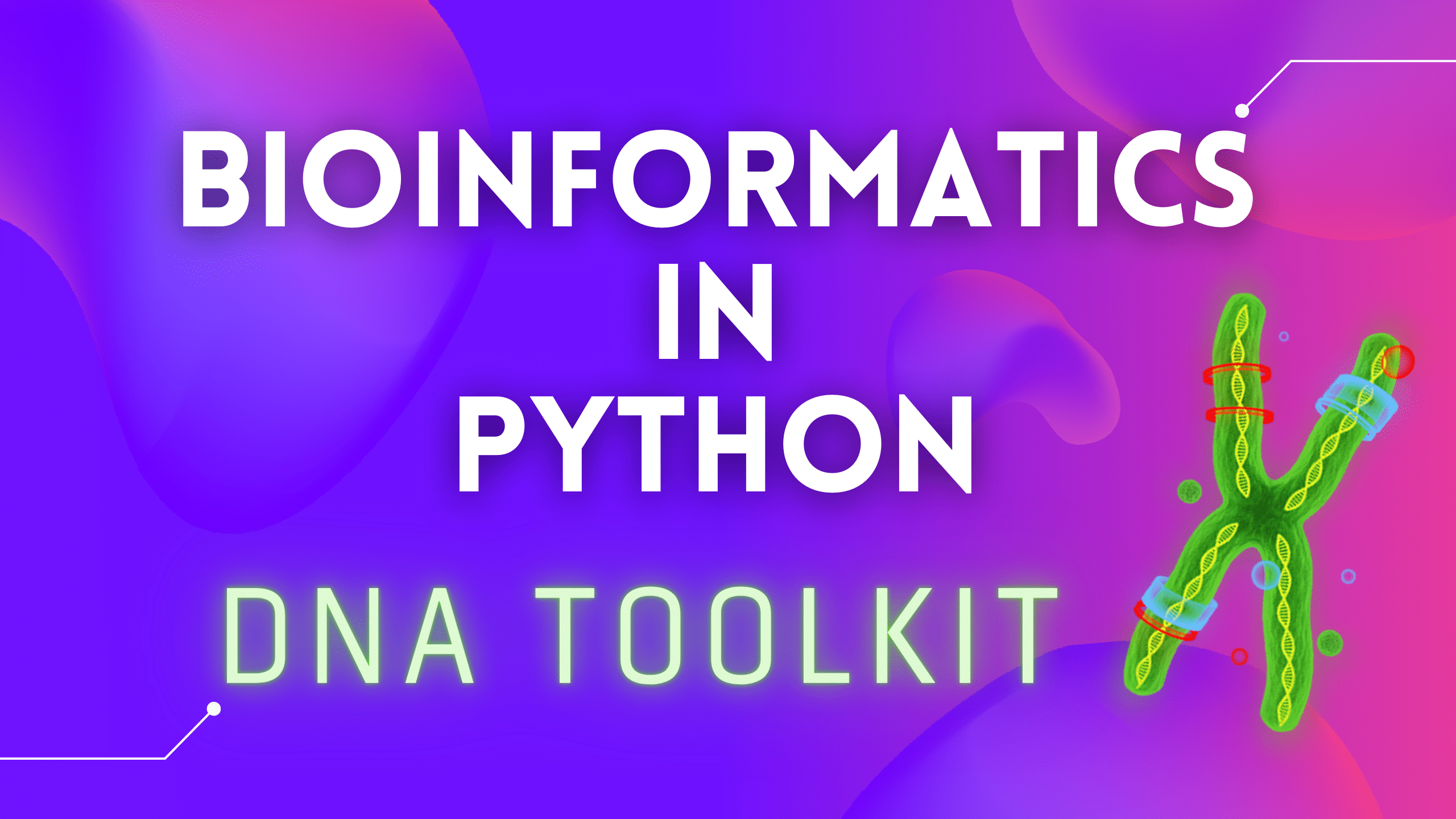# DNA Toolkit Part 4: Translation, Codon UsageBefore we take a look at a Translation function, we need to create a structure to hold the DNA/RNA codon table. We will use a Python dictionary as it is just perfect for holding multiple Keys, that have the same value. Let’s add this to our `structures.py` file:

```DNA_Codons = {
# 'M' - START, '_' - STOP
"GCT": "A", "GCC": "A", "GCA": "A", "GCG": "A",
"TGT": "C", "TGC": "C",
"GAT": "D", "GAC": "D",
"GAA": "E", "GAG": "E",
"TTT": "F", "TTC": "F",
"GGT": "G", "GGC": "G", "GGA": "G", "GGG": "G",
"CAT": "H", "CAC": "H",
"ATA": "I", "ATT": "I", "ATC": "I",
"AAA": "K", "AAG": "K",
"TTA": "L", "TTG": "L", "CTT": "L", "CTC": "L", "CTA": "L", "CTG": "L",
"ATG": "M",
"AAT": "N", "AAC": "N",
"CCT": "P", "CCC": "P", "CCA": "P", "CCG": "P",
"CAA": "Q", "CAG": "Q",
"CGT": "R", "CGC": "R", "CGA": "R", "CGG": "R", "AGA": "R", "AGG": "R",
"TCT": "S", "TCC": "S", "TCA": "S", "TCG": "S", "AGT": "S", "AGC": "S",
"ACT": "T", "ACC": "T", "ACA": "T", "ACG": "T",
"GTT": "V", "GTC": "V", "GTA": "V", "GTG": "V",
"TGG": "W",
"TAT": "Y", "TAC": "Y",
"TAA": "_", "TAG": "_", "TGA": "_"
}```

We are going to use a short, one letter notation instead of a three letter notation, but you can change that for your protect if needed. You could also add a switch to your function to choose which notation you want.

`M` will be a Start codon and `_` will be a Stop codon.

A link to a `structures.py` file on GitLab here, in a case if you want to copy it.

More on codon tables here.

If you are not familiar with DNA/RNA codons, I suggest watching this video:

Now let’s implement a small function, that uses a list comprehension and a `for` loop to read three nucleotides at a time. Codons are basically nucleotide triplets:

We need to read three nucleotides at a time and match them against our `DNA_Codons` dictionary, and that will return an amino acid. That way we can build our amino acid chain also called a polypeptide chain that we can try assembling a protein from. We will look at protein assembly in our next article.

Let’s add this function to our `dna_toolkit.py` file:

```def translate_seq(seq, init_pos=0):
"""Translates a DNA sequence into an aminoacid sequence"""
return [
DNA_Codons[seq[pos:pos + 3]]
for pos in range(init_pos, len(seq) - 2, 3)
]```

If we run this function against this sequence:

``TTGCTAGGATGAGTCGCGAGGTTT``

List comprehension will generate this:

``````TTG - L
CTA - L
GGA - G
TGA - _
GTC - V
GCG - A
AGG - R
TTT - F
['L', 'L', 'G', '_', 'V', 'A', 'R', 'F']``````

This function also has a second parameter, `init_pos`. This will be used in our future functions when we will be generating reading frames. This will allow to start reading codons from any poison on the DNA string. We will come back to that.

Now let’s write a function that computes codon usage, provided an amino acid and a DNA sequence. The result will be a dictionary, where keys will be codons and values will represent the percentage of usage for each of those codons.

Note that since the genetic code is redundant, there will be repeated values, i.e. a single amino acid will be encoded by different codons. This is normally called the codon usage, and provides interesting statistics when applied to the genes of different species.

Excerpt from “Bioinformatics algorithms. Design and implementation” book.

Wikipedia has a very nice article on Codon usage bias here.

```def codon_usage(seq, aminoacid):
"""Provides the frequency of each codon encoding a given aminoacid in a DNA sequence"""
tmpList = []
for i in range(0, len(seq) - 2, 3):
if DNA_Codons[seq[i:i + 3]] == aminoacid:
tmpList.append(seq[i:i + 3])

freqDict = dict(Counter(tmpList))
totalWight = sum(freqDict.values())
for seq in freqDict:
freqDict[seq] = round(freqDict[seq] / totalWight, 2)
return freqDict```

This function does just three things: scans a sequence and looks for an amino acid we specified, accumulates all found instances in a list, and then calculates a number of found sequences.

So let’s look at the example. If we pass the following DNA sequence to our function:

`seq = `TTGCTAGGATGAGTCGCGAGGTTTCTTCACGCCTTTCTTAGTGACTGGTA`aminoacid = `'L'``

Lines `4-6` of our code will generate this list of codons:

``['TTG', 'CTA', 'CTT', 'CTT']``

and store it in `tmpList` (temporary list).

We can see that `'L'` (Leu/Leucine) is present 4 times (marked in bold):

`|`TTG|CTA|GGA|TGA|GTC|GCG|AGG|TTT|CTT|CAC|GCC|TTT|CTT|AGT|GAC|TGG|TA``

Based on that, line `8` will create a dictionary that looks like this:

``{'TTG': 1, 'CTA': 1, 'CTT': 2}``

This is because there are six different codons that code for `L` and we have 3 in our example sequence.

Line `9` will sum up all values (1 + 1 + 2) to get `4`

And finally, lines `10-11` will loop through that `freqDict` (frequencies dictionary) and devide all values by `4`. Now our dictionary will look like this:

``{'TTG': 0.25, 'CTA': 0.25, 'CTT': 0.5}``

Alright. Let’s add two more outputs to our `main.py` file:

```print(
f' + Aminoacids Sequence from DNA: {translate_seq(DNAStr, 0)}\n')

print(
f' + Codon frequency (L): {codon_usage(DNAStr, "L")}\n')```

If we run everything we programmer so far, we should see something like this:

```Sequence: TCTCCTCTACGATCTATGTTTTTTTATAAGTGCCTCCTAGACTTACTCCG

 + Sequence Length: 50

 + Nucleotide Frequency: {'A': 9, 'C': 14, 'G': 6, 'T': 21}

 + DNA/RNA Transcription: UCUCCUCUACGAUCUAUGUUUUUUUAUAAGUGCCUCCUAGACUUACUCCG

 + DNA String + Complement + Reverse Complement:
5' TCTCCTCTACGATCTATGTTTTTTTATAAGTGCCTCCTAGACTTACTCCG 3'
||||||||||||||||||||||||||||||||||||||||||||||||||
3' AGAGGAGATGCTAGATACAAAAAAATATTCACGGAGGATCTGAATGAGGC 5' [Complement]
5' CGGAGTAAGTCTAGGAGGCACTTATAAAAAAACATAGATCGTAGAGGAGA 3' [Rev. Complement]

 + GC Content: 40%

 + GC Content in Subsection k=5: [60, 40, 40, 20, 0, 20, 60, 60, 20, 80]

 + Aminoacids Sequence from DNA: ['S', 'P', 'L', 'R', 'S', 'M', 'F', 'F', 'Y', 'K', 'C', 'L', 'L', 'D', 'L', 'L']

 + Codon frequency (L): {'CTA': 0.4, 'CTC': 0.4, 'TTA': 0.2}```